[Contents]

## 1  Basic Notions of Grounding and Shielding

The ways that noise can couple into a circuit can be classified as

• resistive coupling,
• capacitive coupling, and
• inductive coupling.

## 2  Resistive Coupling

Figure 1 shows a circuit that follows basic sound design principles. There is minimal resistive coupling.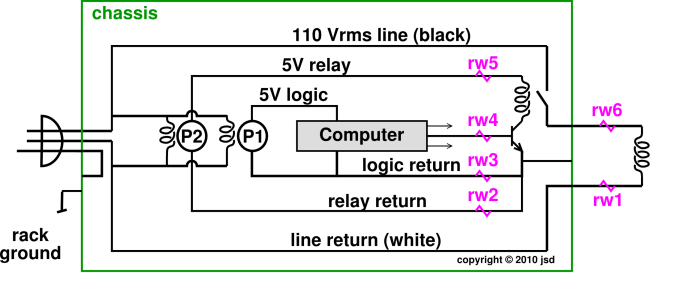Figure 1: Single Point Grounding; Negligible Resistive Coupling

Some salient features of this circuit include:

• Single-point grounding. There are three separate current loops, which are tied together at one and only one point, near the emitter of the driver transistor
• Power supply P1 is transformer isolated from the line.
• Power supply P2 is transformer isolated from the line.
• The computer and other logic is relay isolated from the line.
• The chassis is “grounded” to the third wire i.e. green wire of the power plug.
• The chassis is “grounded” to the rack.
• Circuit ground is tied to the chassis at one and only one point.
• The cold side of the power line is not connected to the rack or the chassis. (It could easily be several volts different from local ground.)

This is not claimed to be an advanced ultra-low-noise design. This is just the basic price of admission.

Non-experts sometimes think that the low side of the power supply is the natural place where things should be grounded, but in figure 1 the one place where all the current loops are tied together is way over on the right, at the emitter of the driver transistor.

In contrast, figure 2 shows a circuit that suffers from a nasty resistive coupling.The problem here is that the heavy current that flows through the relay coils causes an IR drop in (rw2 ∥ rw3). This causes some of the logic levels to be referenced to an unreliable ground level, leading to intermittent logic errors.

A similar problem can be seen in figure 3.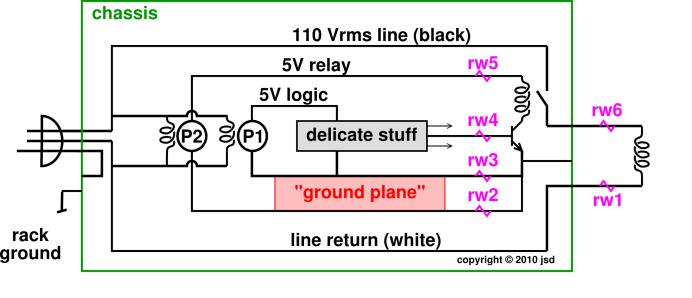Figure 3: Bad: Resistive Coupling ... Cloaca Major

Non-experts sometimes think that if the have something called a “ground plane” they can use it as some sort of cloaca major such that they can flush whatever current they like into it without worrying. Having a ground plane is fine if you use it properly. A ground plane works best if it is an equipotential. It is an equipotential if and only if there is negligible current flowing through it. Connecting the ground plane to logic return at one point is sufficient to guarantee no large currents in it. The power return buses need to be managed carefully and separately, as in figure 4.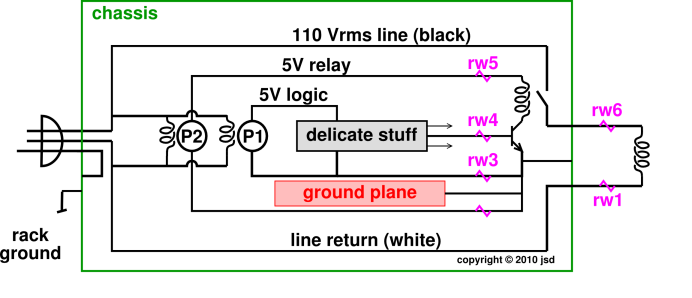Figure 4: Ground Plane with Single-Point Grounding

## 3  Measuring φ•

A quick way to make a rough estimate of the time-dependent magnetic field is shown in figure 5. All you need is an oscilloscope and a loop of wire.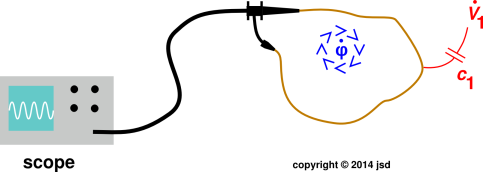Figure 5: Unshielded Loop Antenna

This setup is not ideal, because if there are large time-dependent electric fields, they can couple to the circuit via parasitic capacitances (such as c1). Depending on the resistance of the measuring circuit, this can lead to parasitic IR voltages that mess up the measurement of φ.

If you want a more accurate measurement of φ, you can improve the circuit, as shown in figure 6. In particular, build a small box to which you can attach a piece of standard coax.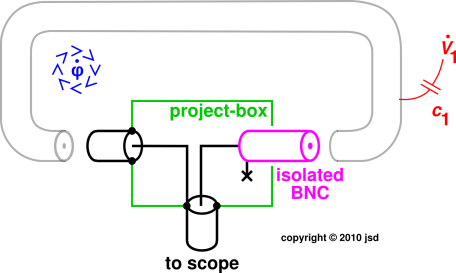Figure 6: Shielded Loop Antenna

Start with a small metal “project box” as diagrammed in green. Add three BNC connectors. Two of them are regular non-isolated “self-grounding” BNC connectors (shown in black) such that the shield is connected to the metal box at these points. The third connector is an isolated BNC (shown in magenta) that leaves the shield completely open-circuited at this point, as indicated by the × in the figure. Wire things up as shown.

You can keep the little box in your bag of tricks. When it comes time to use it, connect a piece of coax to it, as shown in gray in the diagram. The φ will induce a voltage in the inner conductor of the coax, which you can observe using a scope.

The φ will also induce a voltage in the outer conductor of the gray coax, but you don’t care, because it does not appreciably couple to the thing you are measuring.

Note that grounding the outer conductor at point × would be a disaster. It would create a shorted turn, i.e. a one-turn shorted secondary winding. The φ would induce a humongous current in this loop, possibly on the order of amps. In accordance with Lenz’s law this could significantly interfere with what you’re trying to measure.

The reason why you might want a coax (as opposed to the unshielded wire shown in figure ??) is so that capacitively coupled interference, as represented by c1, will be captured by the outer conductor, i.e. the shield. This injects a few microamps of noise into the shield, but we don’t care, because gets drained via a low-impedance path to ground, and does not appreciably couple to the thing we are measuring.

## 4  Ground Loops and Some Ways of Eliminating Them

Figure 7 is an ordinary diagram of an ordinary analog subtractor circuit. This is the sort of diagram you might find in an introductory textbook. This circuit may or may not be something you are interested in per se. However, as we shall see, it is representative of a wider class of circuits, and allows us to explore a number of fundamental grounding and shielding concepts.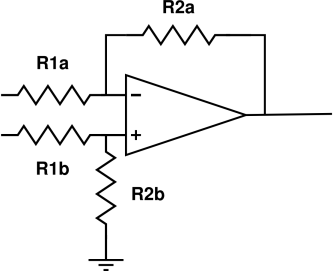Figure 7: Plain Subtractor Circuit

Figure 7 is a fancier diagram version of figure 7. In some over-idealized sense it pertains to the “same” circuit, but depicts quite a number of additional details, including:

• The input and output connections are made via coax cables, and are included in the diagram.
• The parasitic resistance of the coax outer conductor is taken into account, and considered an element of the circuit, represented by r8a and r9a. Similarly the parasitic resistance of the inner conductor is represented by r8b and r9b (not shown in this diagram).
• The chassis is considered an element of the circuit, represented by the dashed red line.
• Various parasitic capacitances are considered elements of the circuit, represented by c1 through c5.
• It is recognized that the concept of “ground” has problems. In particular, “ground” here is not the same as “ground” there. This is diagrammed using two dissimilar symbols for ground.
• The ambient changing magnetic flux φ is shown explicitly. This couples to various circuit elements (especially long cables) via parasitic mutual inductance. This is what causes the dreaded phenomenon known as “ground loops”.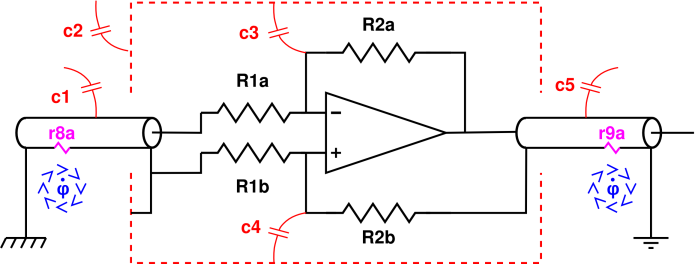Figure 8: Differential Driver, Elementary Grounding and Shielding Analysis
[Contents]